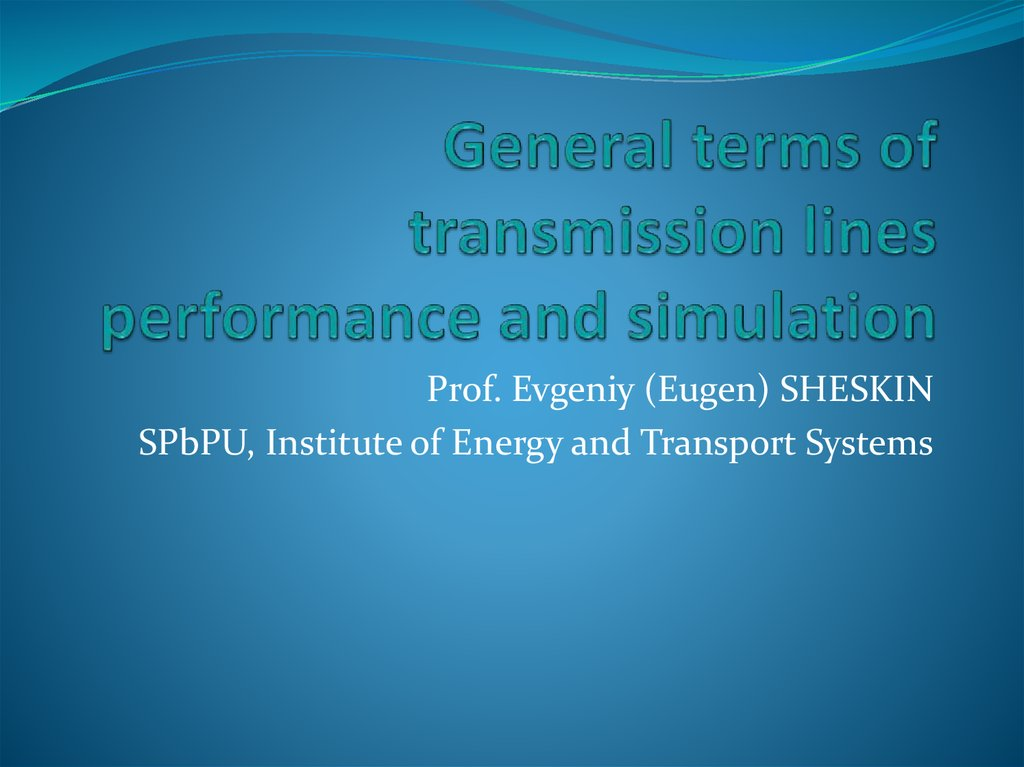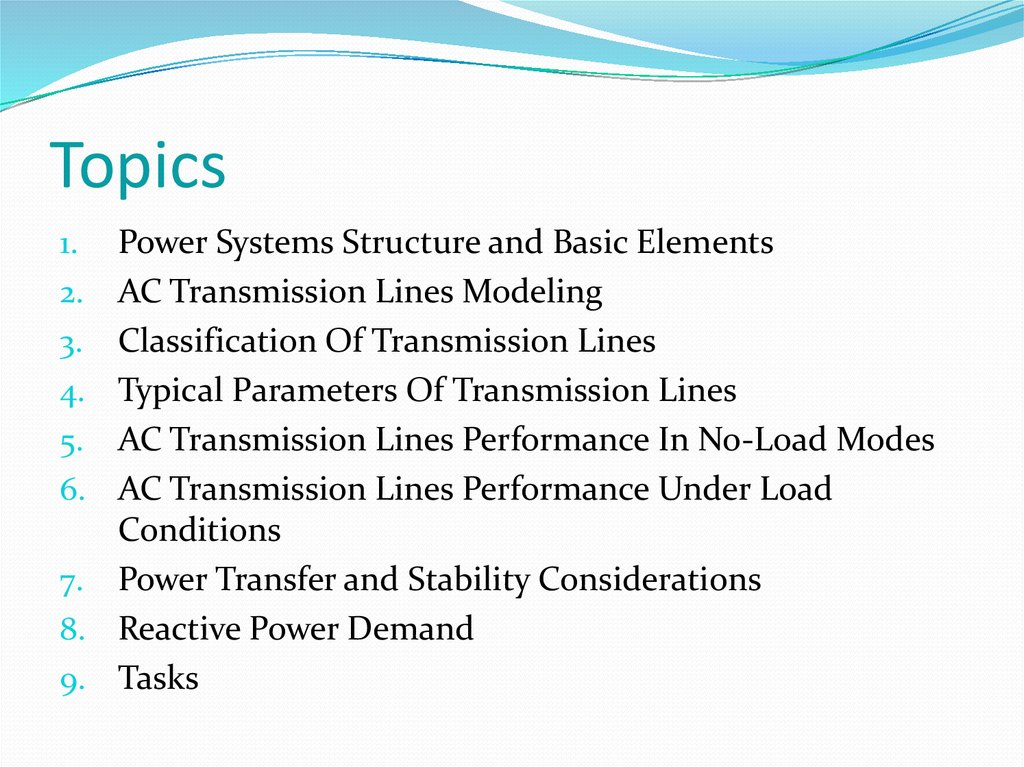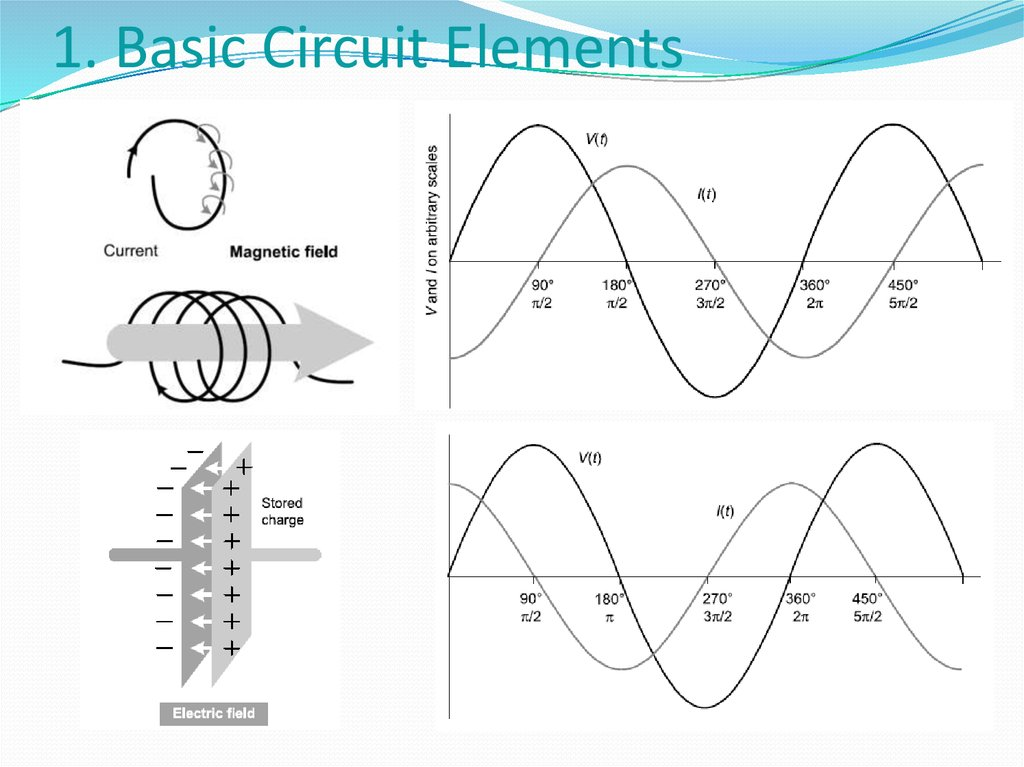# General terms of transmission lines performance and simulation

## 1. General terms of transmission lines performance and simulation

Prof. Evgeniy (Eugen) SHESKIN
SPbPU, Institute of Energy and Transport Systems

## 2. Topics

1.
2.
3.
4.
5.
6.
7.
8.
9.
Power Systems Structure and Basic Elements
AC Transmission Lines Modeling
Classification Of Transmission Lines
Typical Parameters Of Transmission Lines
AC Transmission Lines Performance In No-Load Modes
AC Transmission Lines Performance Under Load
Conditions
Power Transfer and Stability Considerations
Reactive Power Demand

## 4. 1. Phasor Notation

sinusoidally varying voltage is
represented as an arrow of
constant length, spinning around
at the constant frequency ω;
we can ignore this circular
spinning to the extent that it will
be the same for all quantities, and
they are not spinning in relation
to each other (only when f =
const!).

Main elements:
1. Generators;
2. Transformers;
3. Transmission
lines.

## 6. 1. Control Structure

Things we can control:
- Power flows;
- System frequency;
- Node voltages.

## 7. 2. AC Transmission Lines Modeling

To develop performance equations and models for
transmission lines;
To examine the power transfer capabilities of
transmission lines as influenced by voltage, reactive
power, and system stability considerations;
To examine factors influencing the flow of active
power and reactive power through transmission
networks;
To describe analytical techniques for the analysis of
power flow in transmission systems.

## 8. 2. AC Transmission Lines Modeling

Series Resistance (R). The resistances of lines
accounting for stranding and skin effect are
determined from manufacturers’ tables.
Shunt Conductance (G). The shunt conductance
represents losses due to leakage currents along
insulator strings and corona. In power lines, its
effect is small and usually neglected.
Series Inductance (L). The line inductance
depends on the partial flux linkages within the
conductor cross section and external flux
Shunt Capacitance (C). The potential difference
between the conductors of a transmission line
causes the conductors to be charged; the charge
per unit of potential difference is the capacitance
between conductors

## 9. 2. AC Transmission Lines Modeling

AC
transmission line
tower
construction
defines
its
electric
parameters.
AC transmission line
electric
parameters
define its performance
in various under-voltage
conditions.

## 11. 2. AC Transmission Lines Modeling

The constant Zc is called the characteristic impedance and γ
is called the propagation constant.
The constants у and Zc are complex quantities. The real part
of the propagation constant у is called the attenuation
constant α, and the imaginary part the phase constant β.

## 12. 2. AC Transmission Lines Modeling

The power delivered by a transmission line when it is
terminated by its surge impedance is known as the natural
• V and I have constant amplitude along the
line.
• V and I are in phase throughout the length
of the line.
• The phase angle between the sending end
and receiving end voltages (currents) is
equal to θ.

## 13. 2. AC Transmission Lines Modeling

We are letting x = l

## 14. 3. Classification of TL by Length

Short lines: lines shorter than about 100 km (60 mi). They
have negligible shunt capacitance, and may be
represented by their series impedance.
Medium-length lines: lines with lengths in the range of
100 km to about 300 km (190 mi). They may be
represented by the nominal π equivalent circuit.
Long lines: lines longer than about 300 km. For such lines
the distributed effects of the parameters are significant.
They need to be represented by the equivalent π circuit.
Alternatively, they may be represented by cascaded
sections of shorter lengths, with each section represented
by a nominal π equivalent.

## 16. 5. Performance of a TL (no-load)

(a) Receiving end is opened (IR=0)
Neglecting losses
Example: 300 km, 500 kV overhead line, sending end at rated
voltage (1 p.u.). Voltage and current profiles?

Voltage profile
Current profile

## 18. 5. Performance of a TL (no-load)

(b) Line connected to sources at both ends
Assuming ES = ER

Voltage profile
Current profile

## 20. 6. Performance of a TL (under load)

For a lossless line
Several fundamental properties of AC
transmission:
• There is an inherent maximum limit
of power that can be transmitted at
• Any value of power below the
maximum can be transmitted at two
different values of VR. The normal
operation is at the upper value, within
narrow limits around 1.0 pu;
• The load power factor has a significant
influence on VR and the maximum
power that can be transmitted.

## 21. 6. Performance of a TL (under load)

(b) Line connected to sources at both ends
As in the no-load case, assume the magnitudes of the source
voltages at the two ends to be equal.
• The midpoint voltage is midway in phase between ES and
E R;
• The power factor at midpoint is unity;
• With PR>PNAT both ends supply reactive power to the line;
with PR<PNAT, both ends absorb reactive power from the
line.

## 22. 7. Power Transfer and Stability Considerations

Let δ be the angle by
Equating real and imaginary parts

## 23. 7. Power Transfer and Stability Considerations

As the load angle is increased, the
transmitted power increases. This is
accompanied by a reduction in the
midpoint voltage Vm and an increase in
the midpoint current Im so that there is an
increase in power. Up to a certain point
the increase in lm dominates over the
decrease of Vm. When the load angle
reaches 90°, the transmitted power
reaches its maximum value. Beyond this,
the decrease in Vm is greater than the
accompanying increase in Im, hence, their
product decreases with any further
increase in transmission angle.

## 24. 8. Reactive Power Demand

1. Using lossless line equations, solve the case for the line with
fixed (known) sending end voltage and shunt reactor with
XR impedance installed at receiving end.
2. Using lossless line equations, solve the case for the line with
fixed (known) sending end voltage and impedance XS and
shunt reactor with XR impedance installed at receiving end.
3. Determine the maximum voltage at line with fixed sending
end voltage, l = 500 km, XS/Zc = 0.3.
4. Determine the necessary ratings of a shunt reactor installed
at the receiving end of a 750 kV, l = 500 km, XS/Zc = 0.3, PSIL=
2000 MW line to ensure UR=1.05Umax (maximum allowable
voltage).
5. Using data from the 4th task (assuming that you have chosen
the reactor), find maximum voltage on the line (value and
coordinate).